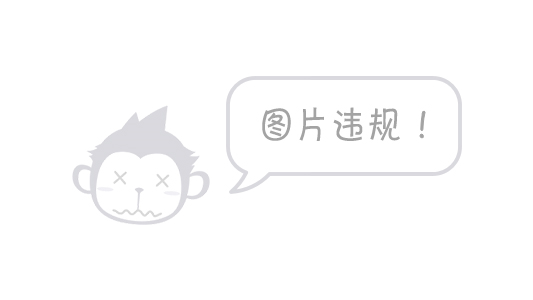# 基于轮廓提取的矫正算法

### 背景

1. 目标识别（匹配、最佳统计分类器、神经网络）
2. 轮廓检测与提取（矩形区域裁剪、灰度化、二值化、ROI）
3. 霍夫变换（Hough），在本次实验中并没有使用上，因为钞票图像有明显的边缘轮廓，而Hough主要用在没有明显边缘轮廓的图像当中，例如文本处理。

#实现过程

## 1、目标检测x 1 = 1011 x_1 = 1011
y 1 = 750 y_1 = 750
x 2 = 2031 x_2 = 2031
y 2 = 3300 y_2 = 3300
x 3 = 2031 x_3 = 2031
y 3 = 3300 y_3 = 3300
x 4 = 2856 x_4 = 2856
y 4 = 2352 y_4 = 2352

## 2、感兴趣区域ROI，将不规则区域填充黑色

Mat mask = Mat::zeros(src.size(),CV_8UC1);
Mat dst;
vector<vector<Point2i>> contours;
vector<Point2i> points;
points.push_back(Point2i(x1, y1));
points.push_back(Point2i(x2, y2));
points.push_back(Point2i(x3, y3));
points.push_back(Point2i(x4, y4));
contours.push_back(points);
drawContours(mask, contours, 0, Scalar::all(255), -1);
src.copyTo(dst, mask);
imwrite("images/result_process.jpg", dst);## 3、轮廓检测与提取

    Mat gray, binImg;
//灰度化
cvtColor(dst,gray, COLOR_RGB2GRAY);
imshow("灰度图", gray);
//二值化
threshold(gray, binImg, 100, 200, CV_THRESH_BINARY);
imshow("二值化", binImg);
contours.clear();
vector<Rect> boundRect(contours.size());
//注意第5个参数为CV_RETR_EXTERNAL，只检索外框
findContours(binImg, contours, CV_RETR_EXTERNAL, CV_CHAIN_APPROX_NONE); //找轮廓
cout << contours.size() << endl;
for (int i = 0; i < contours.size(); i++)
{
//需要获取的坐标
CvPoint2D32f rectpoint;
CvBox2D rect =minAreaRect(Mat(contours[i]));

cvBoxPoints(rect, rectpoint); //获取4个顶点坐标
//与水平线的角度
float angle = rect.angle;
cout << angle << endl;

int line1 = sqrt(pow(rectpoint.y - rectpoint.y,2)+pow(rectpoint.x - rectpoint.x,2));
int line2 = sqrt(pow(rectpoint.y - rectpoint.y,2)+pow(rectpoint.x - rectpoint.x,2));

//面积太小的直接过滤
if (line1 * line2 < 600)
{
continue;
}

//为了让正方形横着放，所以旋转角度是不一样的。竖放的，给他加90度，翻过来
if (line1 > line2)
{
angle = 90 + angle;
}

//新建一个感兴趣的区域图，大小跟原图一样大
Mat RoiSrcImg(dst.rows, dst.cols, CV_8UC3); //注意这里必须选CV_8UC3
RoiSrcImg.setTo(0); //颜色设置为黑色

//对得到的轮廓填充一下
drawContours(binImg, contours, -1, Scalar(255),CV_FILLED);

//抠图到RoiSrcImg
dst.copyTo(RoiSrcImg, binImg);

//再显示一下看看，除了感兴趣的区域，其他部分都是黑色的了
namedWindow("RoiSrcImg", 1);
imshow("RoiSrcImg", RoiSrcImg);

//创建一个旋转后的图像
Mat RatationedImg(RoiSrcImg.rows, RoiSrcImg.cols, CV_8UC1);
RatationedImg.setTo(0);
//对RoiSrcImg进行旋转
Point2f center = rect.center;  //中心点
Mat M2 = getRotationMatrix2D(center, angle, 1);//计算旋转加缩放的变换矩阵
warpAffine(RoiSrcImg, RatationedImg, M2, RoiSrcImg.size(),1, 0, Scalar(0));//仿射变换
imshow("旋转之后", RatationedImg);
imwrite("images/test_temp.jpg", RatationedImg); //将矫正后的图片保存下来
}#if 1
vector<vector<Point> > contours2;
Mat raw = imread("images/test_temp.jpg");
Mat SecondFindImg;
cvtColor(raw, SecondFindImg, COLOR_BGR2GRAY);  //灰度化
threshold(SecondFindImg, SecondFindImg, 80, 200, CV_THRESH_BINARY);
findContours(SecondFindImg, contours2, CV_RETR_EXTERNAL, CV_CHAIN_APPROX_NONE);

for (int j = 0; j < contours2.size(); j++)
{
//这时候其实就是一个长方形了，所以获取rect。
Rect rect = boundingRect(Mat(contours2[j]));
//面积太小的轮廓直接过滤,通过设置过滤面积大小，可以保证只拿到外框。
if (rect.area() < 600)
{
continue;
}
Mat dstImg = raw(rect);
imshow("dst", dstImg);
imwrite("images/test_result.jpg", dstImg);
}
#endif
}# 总结05-07
12-19
10-05
08-11330
01-023789
07-31236
06-277255
12-195170
10-231183
07-194006
01-179311
11-221253
09-141万+
01-163143
08-221万+
04-121万+
©️2020 CSDN 皮肤主题: Age of Ai 设计师:meimeiellie